FanDuel 2019 Stanley Cup Odds
+750
7.5 to 1Bruins
9.4% implied probability

+850
8.5 to 1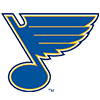Blues
8.4% implied probability

+950
9.5 to 1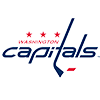Capitals
7.6% implied probability

+950
9.5 to 1Avalanche
7.6% implied probability

+1200
12 to 1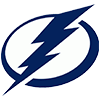Lightning
6.2% implied probability

+1600
16 to 1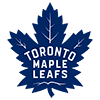Maple Leafs
4.7% implied probability

+1600
16 to 1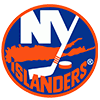Islanders
4.7% implied probability

+1600
16 to 1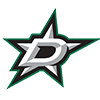Stars
4.7% implied probability

+1700
17 to 1Golden Knights
4.5% implied probability

+1800
18 to 1Predators
4.2% implied probability

+1800
18 to 1Penguins
4.2% implied probability

+1800
18 to 1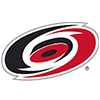Hurricanes
4.2% implied probability

+2000
20 to 1Oilers
3.8% implied probability

+2700
27 to 1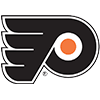Flyers
2.9% implied probability

+2700
27 to 1Coyotes
2.9% implied probability

+3000
30 to 1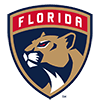Panthers
2.6% implied probability

+3000
30 to 1Canucks
2.6% implied probability

+3000
30 to 1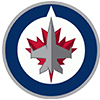Jets
2.6% implied probability

+3600
36 to 1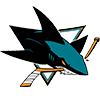Sharks
2.2% implied probability

+3600
36 to 1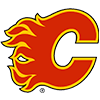Flames
2.2% implied probability

+5500
55 to 1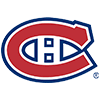Canadiens
1.4% implied probability

+6500
65 to 1Wild
1.2% implied probability

+10000
100 to 1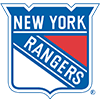Rangers
0.8% implied probability

+10000
100 to 1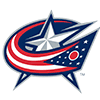Blue Jackets
0.8% implied probability

+10000
100 to 1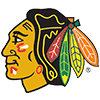Blackhawks
0.8% implied probability

+12000
120 to 1Sabres
0.7% implied probability

+12000
120 to 1Ducks
0.7% implied probability

+18000
180 to 1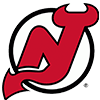Devils
0.4% implied probability

+24000
240 to 1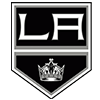Kings
0.3% implied probability

+25000
250 to 1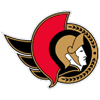Senators
0.3% implied probability

No changes have been recorded yet.
+25000
250 to 1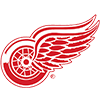Red Wings
0.3% implied probability